Скачать презентацию DAMAGE SPREADING PHASE TRANSITIONS IN A THEMAL

• Количество слайдов: 13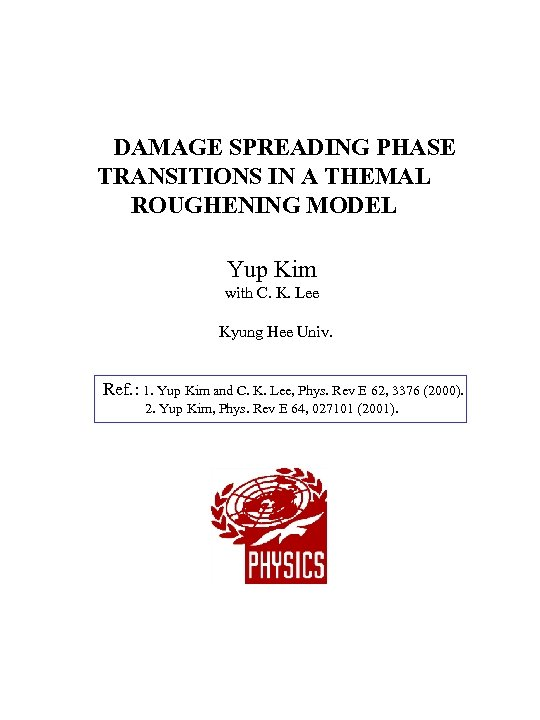DAMAGE SPREADING PHASE TRANSITIONS IN A THEMAL ROUGHENING MODEL Yup Kim with C. K. Lee Kyung Hee Univ. Ref. : 1. Yup Kim and C. K. Lee, Phys. Rev E 62, 3376 (2000). 2. Yup Kim, Phys. Rev E 64, 027101 (2001).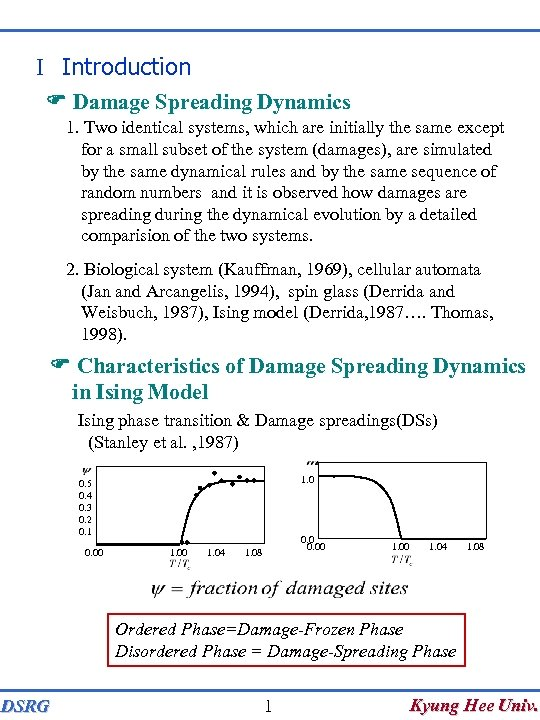I Introduction F Damage Spreading Dynamics 1. Two identical systems, which are initially the same except for a small subset of the system (damages), are simulated by the same dynamical rules and by the same sequence of random numbers and it is observed how damages are spreading during the dynamical evolution by a detailed comparision of the two systems. 2. Biological system (Kauffman, 1969), cellular automata (Jan and Arcangelis, 1994), spin glass (Derrida and Weisbuch, 1987), Ising model (Derrida, 1987…. Thomas, 1998). F Characteristics of Damage Spreading Dynamics in Ising Model Ising phase transition & Damage spreadings(DSs) (Stanley et al. , 1987) 1. 0 0. 5 0. 4 0. 3 0. 2 0. 1 0. 00 1. 04 0. 00 1. 08 1. 00 1. 04 1. 08 Ordered Phase=Damage-Frozen Phase Disordered Phase = Damage-Spreading Phase DSRG 1 Kyung Hee Univ.Kinetic Surface Roughening and Its Scaling relations I Dynamical Self-affinity Surface width W (root-mean-square fluctuation of surface height h(x, t)) ( = correlation length of the surface growth parellel to substrate) L 3 scaling L 2 ln W tb ln (W / La) L 1 ln (t / Lz) ln t DSRG 2 Kyung Hee Univ.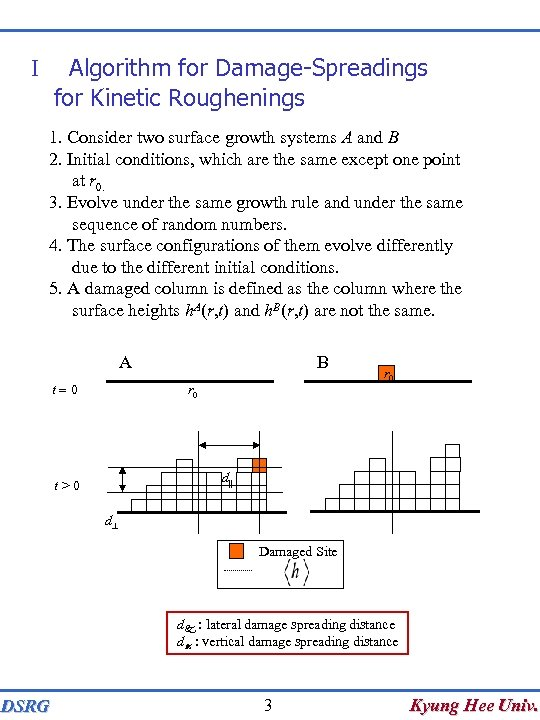Algorithm for Damage-Spreadings for Kinetic Roughenings I 1. Consider two surface growth systems A and B 2. Initial conditions, which are the same except one point at r 0. 3. Evolve under the same growth rule and under the same sequence of random numbers. 4. The surface configurations of them evolve differently due to the different initial conditions. 5. A damaged column is defined as the column where the surface heights h. A(r, t) and h. B(r, t) are not the same. A t=0 B r 0 d|| t>0 d^ Damaged Site d : lateral damage spreading distance d : vertical damage spreading distance DSRG 3 Kyung Hee Univ.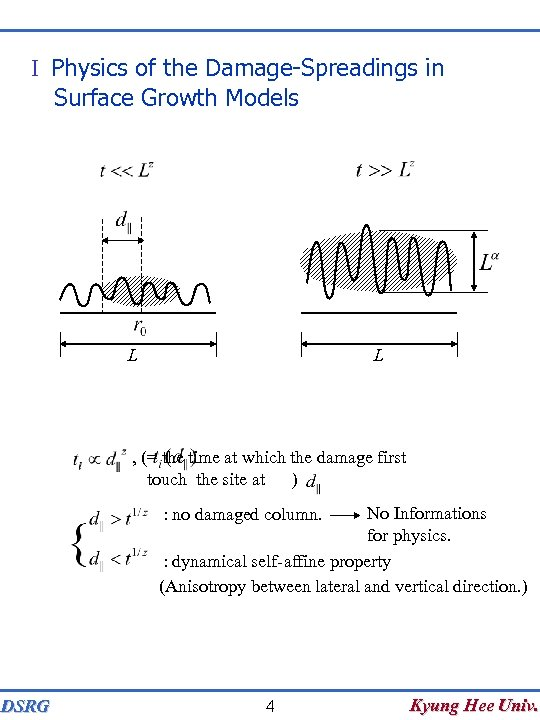I Physics of the Damage-Spreadings in Surface Growth Models L L , (= the time at which the damage first touch the site at ) No Informations for physics. : dynamical self-affine property (Anisotropy between lateral and vertical direction. ) : no damaged column. DSRG 4 Kyung Hee Univ.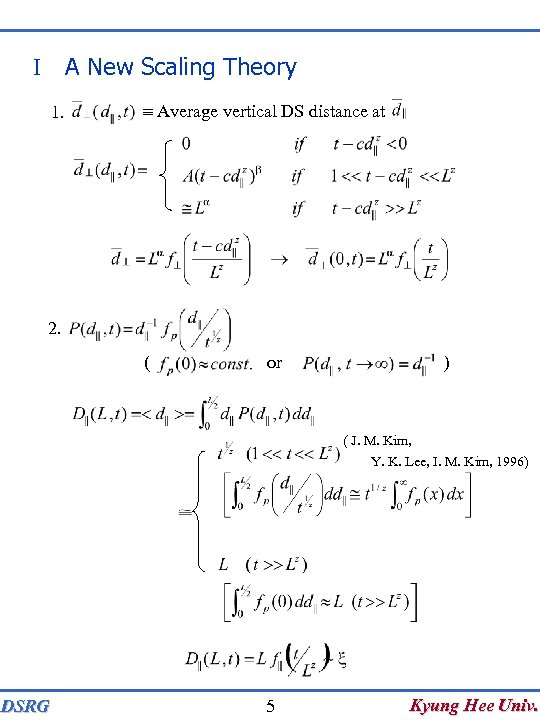A New Scaling Theory I 1. Average vertical DS distance at 2. ( or ) ( J. M. Kim, Y. K. Lee, I. M. Kim, 1996) DSRG 5 Kyung Hee Univ.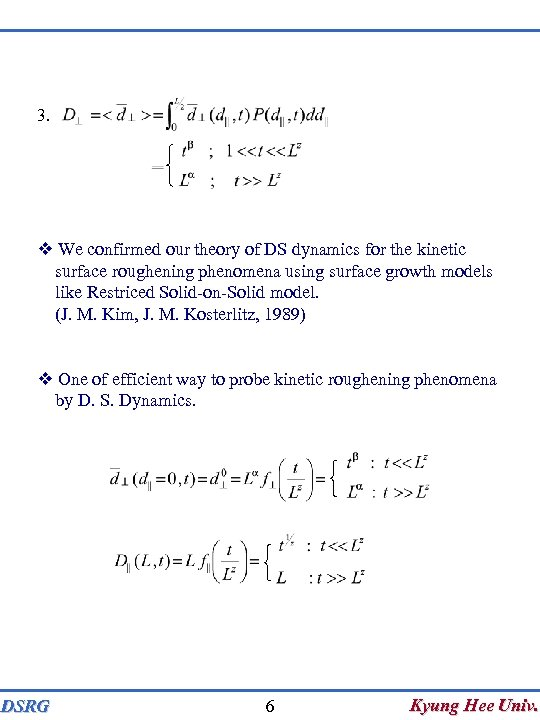3. We confirmed our theory of DS dynamics for the kinetic surface roughening phenomena using surface growth models like Restriced Solid-on-Solid model. (J. M. Kim, J. M. Kosterlitz, 1989) One of efficient way to probe kinetic roughening phenomena by D. S. Dynamics. DSRG 6 Kyung Hee Univ.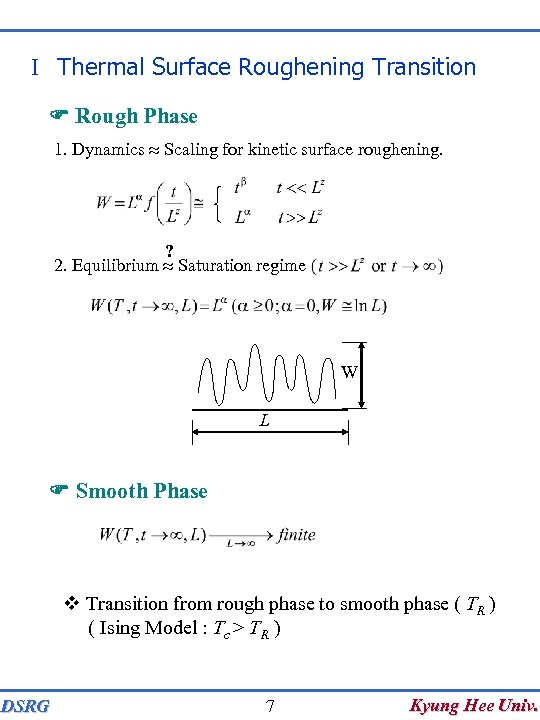I Thermal Surface Roughening Transition F Rough Phase 1. Dynamics Scaling for kinetic surface roughening. ? 2. Equilibrium Saturation regime W L F Smooth Phase Transition from rough phase to smooth phase ( TR ) ( Ising Model : Tc > TR ) DSRG 7 Kyung Hee Univ.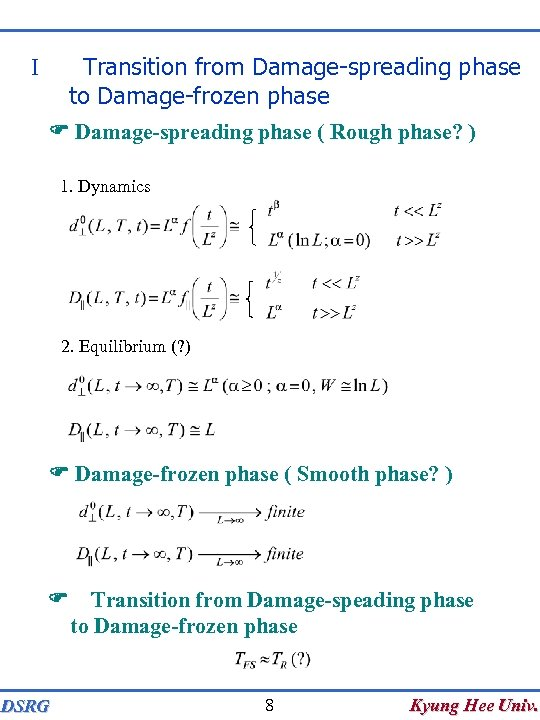Transition from Damage-spreading phase to Damage-frozen phase I F Damage-spreading phase ( Rough phase? ) 1. Dynamics 2. Equilibrium (? ) F Damage-frozen phase ( Smooth phase? ) F Transition from Damage-speading phase to Damage-frozen phase DSRG 8 Kyung Hee Univ.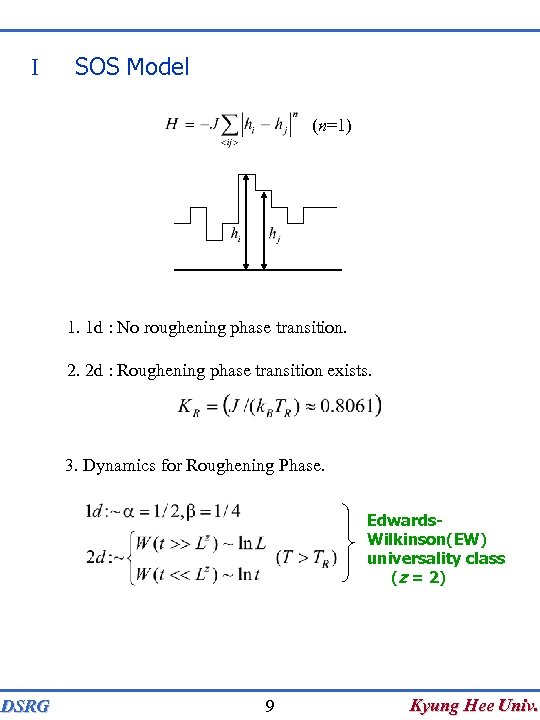I SOS Model (n=1) 1. 1 d : No roughening phase transition. 2. 2 d : Roughening phase transition exists. 3. Dynamics for Roughening Phase. Edwards. Wilkinson(EW) universality class (z = 2) DSRG 9 Kyung Hee Univ.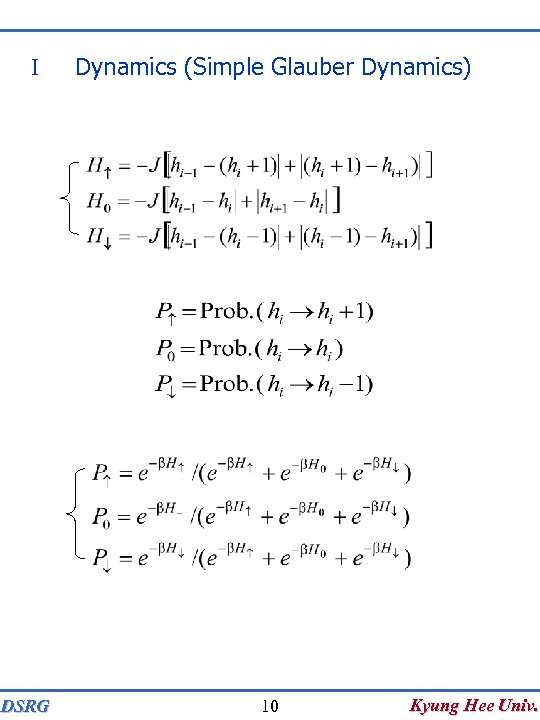I DSRG Dynamics (Simple Glauber Dynamics) 10 Kyung Hee Univ.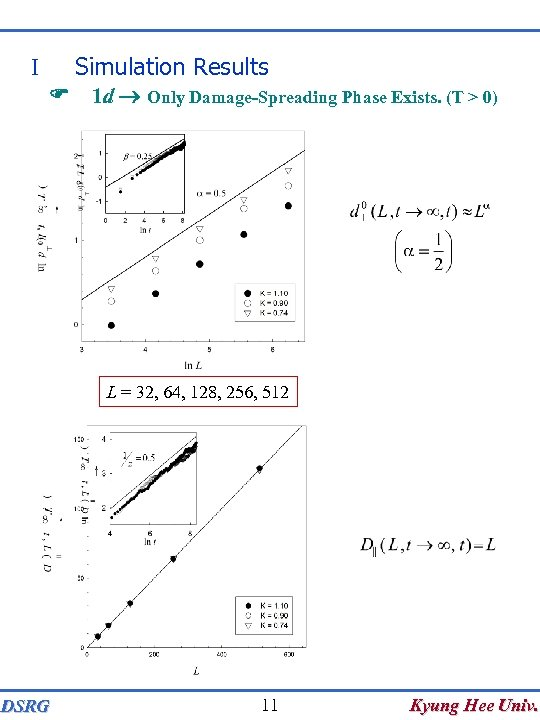Simulation Results I F 1 d Only Damage-Spreading Phase Exists. (T > 0) L = 32, 64, 128, 256, 512 DSRG 11 Kyung Hee Univ.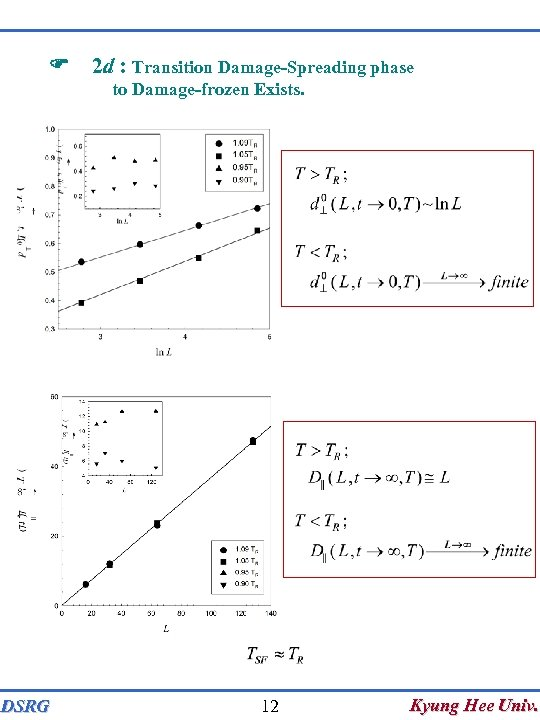F 2 d : Transition Damage-Spreading phase to Damage-frozen Exists. DSRG 12 Kyung Hee Univ.